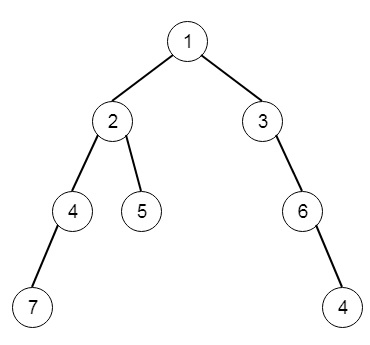# Deepest Leaves Sum in C++

C++Server Side ProgrammingProgramming

Suppose we have a binary tree, we have to find the sum of values of its deepest leaves. So if the tree is like −Then the output will be 15.

To solve this, we will follow these steps −

• Define a map m, and maxDepth
• Define a recursive method solve(), this will take node and level, initially level is 0
• if node is not present, then return
• maxDepth := max of level and maxDepth
• increase m[level] by value of node
• solve(left of node, level + 1)
• solve(right of node, level + 1)
• In the main method, setup maxDepth := 0, then solve(root, 0)
• return m[maxDepth]

## Example(C++)

Let us see the following implementation to get a better understanding −

Live Demo

#include <bits/stdc++.h>
using namespace std;
class TreeNode{
public:
int val;
TreeNode *left, *right;
TreeNode(int data){
val = data;
left = NULL;
right = NULL;
}
};
void insert(TreeNode **root, int val){
queue<TreeNode*> q;
q.push(*root);
while(q.size()){
TreeNode *temp = q.front();
q.pop();
if(!temp->left){
if(val != NULL)
temp->left = new TreeNode(val);
else
temp->left = new TreeNode(0);
return;
}
else{
q.push(temp->left);
}
if(!temp->right){
if(val != NULL)
temp->right = new TreeNode(val);
else
temp->right = new TreeNode(0);
return;
}
else{
q.push(temp->right);
}
}
}
TreeNode *make_tree(vector<int> v){
TreeNode *root = new TreeNode(v);
for(int i = 1; i<v.size(); i++){
insert(&root, v[i]);
}
return root;
}
class Solution {
public:
int maxDepth;
map <int, int> m;
void solve(TreeNode* node, int level = 0){
if(!node)return;
maxDepth = max(level, maxDepth);
m[level] += node->val;
solve(node->left, level + 1);
solve(node->right, level + 1);
}
int deepestLeavesSum(TreeNode* root) {
maxDepth = 0;
m.clear();
solve(root);
//cout << maxDepth << endl;
return m[maxDepth];
}
};
main(){
vector<int> v = {1,2,3,4,5,NULL,6,7,NULL,NULL,NULL,NULL,8};
TreeNode *root = make_tree(v);
Solution ob;
cout << (ob.deepestLeavesSum(root));
}

## Input

[1,2,3,4,5,null,6,7,null,null,null,null,8]

## Output

15